# Dices

We will throw two dice. What is the probability that the ratio between numbers on first and second dice will be 1:2?

p =  0.17 %

### Step-by-step explanation:

$p=\frac{2\cdot 3}{6\cdot 6}=\frac{1}{6}=0.17\mathrm{%}$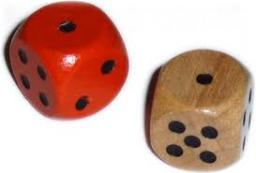Did you find an error or inaccuracy? Feel free to write us. Thank you!Tips to related online calculators
Need help to calculate sum, simplify or multiply fractions? Try our fraction calculator.
Would you like to compute count of combinations?

## Related math problems and questions:

• ThrowWe throw 2 times with 2 dices. What is the probability that the first roll will fall more than sum of 9 and the second throw have sum 3 or does not have the sum 4?
• The diceWhat is the probability of events that if we throw a dice is rolled less than 6?
• Dice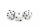How many times must throw the dice, the probability of throwing at least one six was greater than 90%?
• Sum 10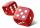What is the probability that two dice throw twice in a row will result the sum of 10?
• Three dice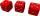What is the probability that when we roll three dice, we roll the numbers 1,2,3?
• One threeWe throw two dice. What is the probability that max one three falls?
• DiceWe throw five times the dice. What is the probability that six fits exactly twice?
• Dices throwsWhat is the probability that the two throws of the dice: a) Six falls even once b) Six will fall at least once
• Ten dices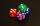When you hit ten dices at the same time you get average 35. How much do you hit if every time you get six, you're throwing the dice again?
• DiceWe throw 10 times a playing dice. What is the probability that the six will fall exactly 4 times?
• Sum on diceWe have two dice. What is the greater likelihood of fall a total sum 7 or 8 ? (write 7, 8 or 0 if the probabilities are the same)?
• Two diceWe roll two dice. What is the probability that the sum of the falling numbers is greater than 3?
• Three dice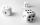When you throw three dice was the sum total of the dice 10. The yellow dice fell one eye more than on the red and brown fell 3 eyes less than red. How many eyes fell on every dice?
• Sum of fall dices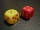What is the probability that the sum of 9 will fall on a roll of two dice? Hint: write down all the pairs that can occur as follows: 11 12 13 14 15. . 21 22 23 24. .. . 31 32. .. . . . . . .. . 66, count them, it's the variable n variable m: 36, 63,. .. .
• AceFrom complete sets of playing cards (32 cards), we pulled out one card. What is the probability of pulling the ace?
• PediatricianPediatrician this month of 20 working days takes 8 days holidays. What is the probability that on Monday it will be at work?
• Six on diceWhat is the probability that when throwing two dice will fall at least one six?